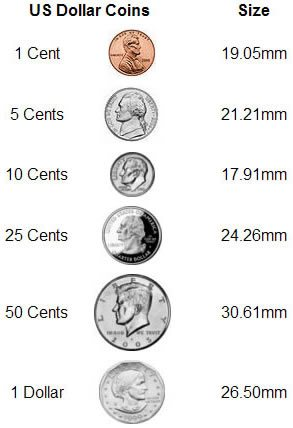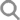تسجيل الدخول تسجيل الاشتراك دعم العملاء متحدث الإنجليزية فقط مجانى بالكامل - الولايات المتحدة الأمريكية & أمب؛ كندا فقط: 1-800-464-1640 دولي: +66-39303404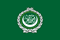USD ابدأ من هنا تسوق بواسطة تسوق بواسطة وصل مؤخرا كابوشون أزواج مجموعات قياسية المساعدة المساعدة عنا
 Frequently Asked Questions (FAQ) / Specific Gravity & Gemstone Density What is the Meaning of "Specific Gravity" for Gemstones? What Exactly Does it Measure?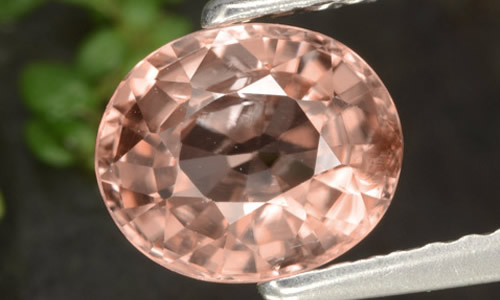Specific gravity is a way to express the relative density of a gemstone. It is measured as the ratio of the density of the gemstone to the density of water, and is expressed as a number that indicates how much heavier the gemstone is compared to an equal volume of water. Scientifically, specific gravity is defined as a ratio of the mass of a given material to the mass of an equal volume of water at 4 degrees centigrade. Most gemstone substances are two to four times denser than an equal volume of water. Specific gravities are expressed in decimal numbers, for example, 4.00 for corundum, 3.52 for diamond and 2.72 for quartz. Zircon is one of the densest gemstones (pictured above); it can have a specific gravity as high as 4.73! To calculate exact specific gravity values, various measuring devices are used. Rough approximations of the specific gravity of lighter stones can be made by using a series of liquids with known specific gravity values. For example, if a stone floats in a liquid with a specific gravity of 4 and sinks in a liquid with a specific gravity of 3, the specific gravity of the stone must lie between these values. See our specific gravity chart for density ratings of the different types of gemstones. Return to FAQ HOME
• نشرت لأول مرة: فبراير-20-2006
• آخر تحديث: سبتمبر-12-2017
• © 2005-2020 جميع الحقوق محفوظة ل gemselect و أى استنساخ (نص أو رسومات) ممنوع منعا باتا.
 أشكال أكثر
أحجار الكريمة شعبية
•  زفير
•  زمرد
•  ياقوت
•  زبرجد أو أكوامارين
•  زركون
•  أوبال
•  توباز
•  تورمالين
•  عقيق
•  جمشت
•  سيترين
•  تنزانيت
جميع الأحجار الكريمة (145)
•  أباتيت
•  أباتيت عين القط
•  أحجار كريمة عين القط
•  أحجار كريمة نجمية
•  أحجار كريمة يشم
•  أزوريت دروزى
•  أغويط عين القط
•  ألماس
•  أم اللؤلؤ
•  أموليت
•  أميترين
•  أوبال
•  أوبال أسود
•  أوبال النار
•  أوبال الهياليت
•  أوبال دوبليت
•  أوبال شيكولاتى اللون
•  أوبال طحلبى
•  أوبال عين القط
•  أوبال فى مصفوفة
•  إسبنيل
•  إنستاتايت
•  إيدوكراز
•  إيوليت
•  اسبكتروليت
•  افينتورين
•  البيترسايت
•  الحجر المعالج
•  السيرافينيت
•  الفلوريت معدن متبلور
•  الكالسيت
•  الكوارتز الغامض
•  الكيانيت
•  النوم ميت
•  الهيدنيت
•  اليشمك
•  امازونيت
•  اندلوسيت
•  بريهانايت
•  بيريت
•  بيريت قوس قزح
•  بيريل
•  بيريل ذهبى
•  تنزانيت
•  توباز
•  توباز إمبراطورى
•  توباز غامص
•  تورمالين
•  تورمالين أخصر
•  تورمالين روبليت
•  جمشت
•  جمشت دروزى
•  جيود عقيق
•  حجر الخرز
•  حجر الدم
•  حجر السربنتين
•  حجر الشمس
•  حجر القمر
•  حجر القمر عين القط
•  حجر القمر قوس قزح
•  حجر القمر نجمة
•  حجرالشمس نجمى
•  دياسبور متغير اللون
•  ديوبسيد كروم
•  ديوبسيد نجمة
•  رودوكروزيت
•  زبرجد
•  زبرجد أو أكوامارين
•  زبرجد عين القط
•  زركون
•  زفير
•  زفير متغير اللون
•  زفير نجمى
•  زمرد
•  سبج أو أوبسيديان
•  سبج ندفة الثلج
•  سفاليرايت
•  سكابوليت
•  سكاتوليت عين القط
•  سكوليسيت
•  سليمانايت
•  سميثسونايت
•  سودالايت
•  سيترين
•  سيفين
•  شارويت
•  صخر الأوبال
•  عقيق
•  عقيق
•  عقيق أبيض أو كالسيدونى
•  عقيق الألماندين
•  عقيق الديمانتويد
•  عقيق السبيسارتيت
•  عقيق المالايا
•  عقيق النار
•  عقيق برتقالى أو كارنيليان
•  عقيق بيروب
•  عقيق تسافوريت
•  عقيق رودوليت
•  عقيق شجيري
•  عقيق غروسوليت
•  عقيق مالى
•  عقيق متغير اللون
•  عقيق نجمى
•  عقيق هيسونيت
•  عين الصقر
•  عين القط أكتينوليت
•  عين القط سيليمانايت
•  عين النمر
•  غرانديديريت
•  فارسيسايت
•  فلوريت متغير اللون
•  فيروز
•  كريزوبرايس
•  كريزوبيريل
•  كريزوكولا
•  كوارتز
•  كوارتز الفراولة
•  كوارتز روتيل
•  كوارتز زهرى
•  كوارتز زهرى نجمة
•  كوارتز سموكى
•  كوارتز عين القط
•  كوارتز ليمونى نجمى
•  كوارتز مع مرقشيتا
•  كورنيروبين
•  كونزيت
•  لؤلؤ
•  لابرادوريت
•  لابرادوريت أنديسين
•  لاريمار
•  لازورد
•  ماو-سيت-سيت
•  مرجان
•  مرجان حجرى
•  مصفوفة عين النمر
•  ملاكيت
•  مورجانيت
•  هيماتيت حجر الدم
•  هيميمورفيت دروزي
•  ياقوت
•  ياقوت الزوزيت
•  ياقوت في فوشسيت
•  ياقوت نجمة
•  يشب أو جاسبر
الفئات الرئيسية
•  وصل مؤخرا
•  مجموعات من الأحجار الكريمة
•  أحجار كريمة قياسية
•  أحجار كريمة بالقطعة
•  أحجار كريمة أعلى درجة
•  مطابقة أزواج
•  أحجار كريمة كابوشون
•  خرز وبريوليت وأحجار مثقوبة
•  أحجار البخت
•  منحوتات الأحجار الكريمة
•  أحجار كريمة فانسى
•  أحجار كريمة نجمية
•  زفير غير مسخن
 دعم العملاء متحدث الإنجليزية فقط مجانى بالكامل - الولايات المتحدة الأمريكية & أمب؛ كندا فقط: 1-800-464-1640 دولي: +66-39303404

وفر المال
لا رسوم شحن للأصناف الإضافية!
\$ 8.90 الشحن في جميع أنحاء العالم

Update Translation

 Current Value New Value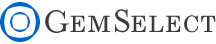X أغلق النافذةX أغلق النافذةX أغلق النافذةالحجم والوزن Gems are always measured in Millimeter (mm) Dimensions are given as; length x width x depth, except for round stones which are; diameter x depth Select gems by size, not by weight! Gem varieties vary in density, so carat weight is not a good indication of size Note: 1ct = 0.2g